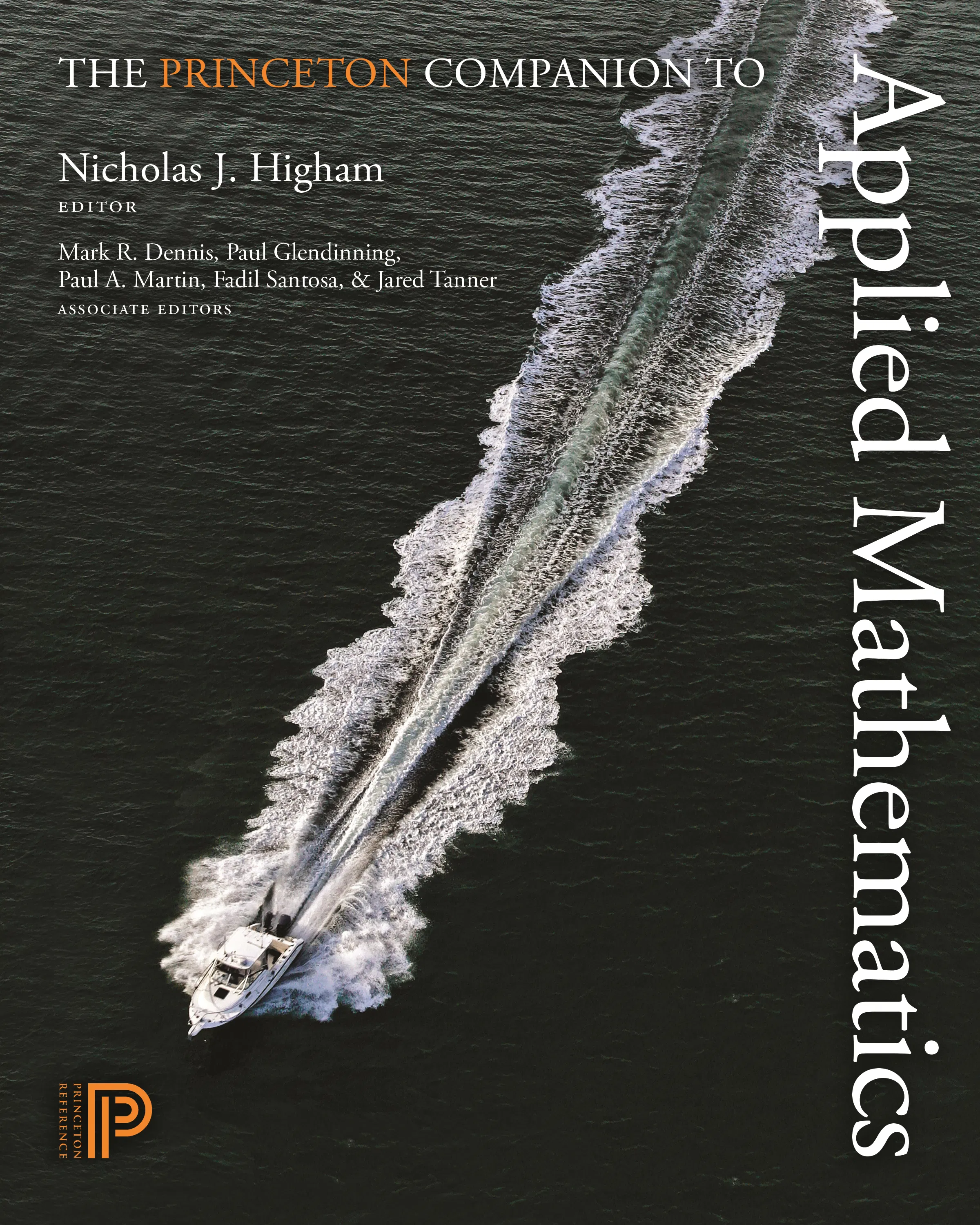#### The Princeton Companion to Applied Mathematics

Nicholas J. Higham

##### ebooks

Many of our ebooks are available for purchase from these online vendors:

Many of our ebooks are available through library electronic resources including these platforms:

# The Princeton Companion to Applied Mathematics

Associate editor
• Mark R. Dennis
• Paul Glendinning
• Paul A. Martin
• Jared Tanner

The must-have compendium on applied mathematics## ebook

ISBN:
Published:
09/15/2015
2016
Illus:
23 color illus. 20 halftones. 160 line illus.

This is the most authoritative and accessible single-volume reference book on applied mathematics. Featuring numerous entries by leading experts and organized thematically, it introduces readers to applied mathematics and its uses; explains key concepts; describes important equations, laws, and functions; looks at exciting areas of research; covers modeling and simulation; explores areas of application; and more.

Modeled on the popular Princeton Companion to Mathematics, this volume is an indispensable resource for undergraduate and graduate students, researchers, and practitioners in other disciplines seeking a user-friendly reference book on applied mathematics.

• Features nearly 200 entries organized thematically and written by an international team of distinguished contributors
• Presents the major ideas and branches of applied mathematics in a clear and accessible way
• Explains important mathematical concepts, methods, equations, and applications
• Introduces the language of applied mathematics and the goals of applied mathematical research
• Gives a wide range of examples of mathematical modeling
• Covers continuum mechanics, dynamical systems, numerical analysis, discrete and combinatorial mathematics, mathematical physics, and much more
• Explores the connections between applied mathematics and other disciplines
• Includes suggestions for further reading, cross-references, and a comprehensive index